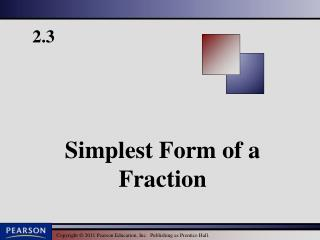# 2.3 - PowerPoint PPT PresentationDownload Presentation2.3

2.3
Download Presentation## 2.3

- - - - - - - - - - - - - - - - - - - - - - - - - - - E N D - - - - - - - - - - - - - - - - - - - - - - - - - - -
##### Presentation Transcript

1. Writing Fractions in Simplest Form Fractions that represent the same portion of a whole are called equivalent fractions. There are many equivalent forms of a fraction. A special form of a fraction is called simplest form. Simplest Form of a Fraction A fraction is written in simplest form or lowest terms when the numerator and denominator have no common factors other than 1. p 129

2. Writing Fractions in Simplest Form Both have a common factor of 2. There are no more common factors. The simplest form of is Alternative method P 129

3. Examples Write in simplest form. a. b. c. p 129

4. Simplest Form Writing a Fraction in Simplest Form To write a fraction in simplest form, write the prime factorization of the numerator and the denominator and then divide both by all common factors. p 129

5. Examples Write in simplest form. a. b. c. p 130

6. Writing Fractions in Simplest Form No common Factors P 130

7. Example Determine whether are equivalent. Simplify each fraction. Since both of the simplified fractions are the same, they are equivalent. p 133

8. Determining Whether Two Fractions Are Equivalent EXAMPLE Determine whether are equivalent. SOLUTION Since both have a common simplified form of they are equivalent. P 132

9. Determining Whether Two Fractions Are Equivalent EXAMPLE Determine whether are equivalent by cross multiplying. SOLUTION Since the cross products are not equal, are not equivalent. P 133

10. Determining Whether Two Fractions Are Equivalent Practice Problem 8 Determine whether are equivalent. SOLUTION Since both have a common simplified form of they are equivalent. P 132

11. Determining Whether Two Fractions Are Equivalent Do Practice Problem 8 Again Determine whether are equivalent by cross multiplying. SOLUTION Since the cross products is equal, are equivalent. P 132

12. Determining Whether Two Fractions Are Equivalent Practice Problem 9 Determine whether are equivalent. SOLUTION Since they do not have a common simplified form they are not equivalent. P 133

13. Determining Whether Two Fractions Are Equivalent Do Practice Problem 9 Again Determine whether are equivalent by cross multiplying. SOLUTION Since the cross products are not equal, they are not equivalent. P 133

14. Solving Problems by WritingFractions in Simplest Form EXAMPLE There are 5280 feet in a mile. What fraction of a mile is represented by 2640. SOLUTION Both 2640 and 5280 have a common factor of 2640. The fraction of a mile represented by 2640 is P 133

15. Solving Problems by WritingFractions in Simplest Form Practice Problem 10 (alternate) Eighty pigs were used in a recent study of olestra, a calorie-free fat substitute. A group of 12 of these pigs were fed a diet high in fat. What fraction of the pigs were fed the high-fat diet in this study? Write your answer in simplest form. SOLUTION Both 12 and 80 have a common factor of 4. The fraction for the group of 12 pigs is represented by P 133

16. DONE

17. Example Determine whether are equivalent by cross multiplying. Since 22 ≠20, then p 133